Do you like the article?
Share it with others —
Use new possibilities of MetaTrader 5

#### Similar articles# Combinatorics and probability theory for trading (Part II): Universal fractal8 6821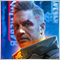### Introduction

In the previous article, we discussed the basics of probability theory which will assist us in understanding the specific features of using fractal functions for trading tasks. As a continuation of this topic, I will show some self-sufficient fractal functions which can describe all required pricing processes. We will try to generalize and simplify them, as well as create formulas to answer various questions which lack clear quantitative estimates and unambiguous answers.

### Assessing the possibilities of using fractals in trading

Let us continue the topic by summarizing the results of the previous article and by presenting the material in a more compact and universal form. Do you remember the example of constructing a fractal from the previous article? Such a fractal is of little practical use, but in this case we are mainly interested in the structure construction rules that we have determined. As it turned out, these rules can be applied to three types of fractals:

1. Fractal with symmetrical borders
2. Fractal with asymmetric borders
3. Fractal with upper or lower border

Such fractals can be applied to describe the following processes:

• Accelerated imitation of trading with the ability to evaluate the probabilities of various scenarios, taking into account deposit restrictions (since the lower border can symbolize the deposit level at which further trading will be impossible)
• Estimation of the average number of steps within a fractal (for example, you can estimate how many orders we have on average before we get either the desired profit or loss)
• Estimation of the total average values for each step (for example, you can calculate the average position holding time, based on statistics for a smaller position, i.e. a position with smaller stop levels in points or in price difference
• Evaluation of the profitability of options based on a single bordered fractal
• Other capabilities

### Theoretical basis for building a universal fractal

Let's use the construction rules that we derived in the previous article, and supplement them to understand how a fractal is constructed. In addition, I have found a small mistake in my formulas, due to which downward or upward asymmetrization of borders was impossible. The derived formulas turned out to be correct, and thus they work for absolutely any fractal. Actually, this is a function for implementing absolutely any fractal. All possible fractals are a special case of a general fractal. If we take the three fractal types defined above, the conditions of the general fractal for the implementation of these three special cases will be as follows:

1. m = n & [ m > s & n > s ]
2. ( m > n || n > m )  & [ m > s & n > s ]
3. ( m > S && n <= S ) || ( n > S && m <= S )

Schematically, these three types of fractals look like this: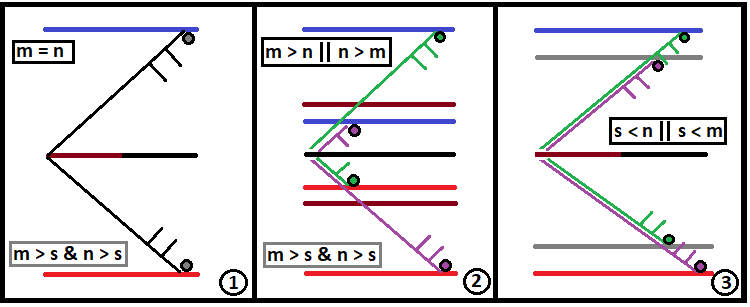Ideally, "S" should tend to infinity. The following variables were not described in my previous article. I will provide the relevant descriptions here to get a complete picture of how to use the general formula to get the special cases. A fractal is a function that works on the principle of a chain reaction, as in an atomic bomb. If the set chain reaction is too deep, the computer may fail to cope with such massive calculations. If the case is not particularly critical, it will simply count for a very long time — minutes, hours or even days. To correctly launch a chain reaction in a fractal, we should find two fundamental values:

• Half - half of the channel width
• Middle - the "U" value which corresponds to the middle line

The Half value can be easily calculated for all the three cases that we determined in the previous article: it is the arithmetic mean of m and n:

• Half = ( n + m ) / 2

To implement the second value, we will have to use three logic variants. However, the first and the second variants can be combined into one. Thus, we have two variants:

1. n >= m
2. n < m

Next, given that the "U" axis is directed up and the n value is the upper part of the channels, while m is the lower part, we get two ratios for two cases with possible m and n:

1. Middle = Half - m
2. Middle = - ( Half - n )

These values will be passed to the fractal function for internal use, because the internal branching logic described in the previous article cannot be implemented without them. The function prototype is as follows:

• double Fractal(double Half, double Middle, int m, int n, int s,double p,int S, int U, double P)

Thus, we need to pass three mandatory values for the correct fractal start:

1. Half - half of the channel width
2. Middle - the "U" value which corresponds to the middle line
3. m - the number of steps to the lower border
4. n - the number of steps to the upper border
5. s - the maximum allowable number of steps in any direction for a single chain

Other values are indicated by capital letters to show that these values are dynamic and that they will be different on different fractal levels. Here is their definition:

• S - the number of steps accumulated in the current chain of probabilities; to be passed to the next fractal level
• U - current distance between the chain start point and its end; to be passed to the next fractal level
• P - accumulated product of probabilities of the entire chain based on the Bernoulli scheme; to be passed to the next fractal level

So, for a correct start of the fractal, we should input the following values into the function:

• S = 0 (since it is a start, so there have been no steps yet)
• U = 0 (for the same reason)
• P = 1 (since it is a zero chain and all next steps should make up a complete group)

To finish developing the general rules for fractals that simulate pricing or trading, let's briefly rewrite the rules that were obtained in the previous article. These rules are used inside the fractal. The rules are based on several formulas for the same steps:

• f = u + d  — it is the number of steps of the future combinations tree (the distance is determined by the distance to the nearest border of the fractal range)
• s = u - d  — the number of final steps, expressed in terms of falling and rising segments

We have determined that we will loop through "u". In addition, we will use the "s' value as the new "U", which will be passed to the next fractal level, if the number of remaining steps supports it. For this purpose, we need to define a formula for "u" which does not contain "d". To do this, express "d" from the first equation and substitute it into the second one:

• s = 2*u - f

This value could also be used as the new value of "U" to pass further if the current value were equal to zero. So, we need to add this "s" to "U" to obtain the value that should be passed further:

• NewU = s + U  - our new "U" to pass to the next fractal level

As already defined in the previous article, this expression takes three possible values, based on the three possible values of the number "f". I have revised a diagram from the previous article to illustrate the idea: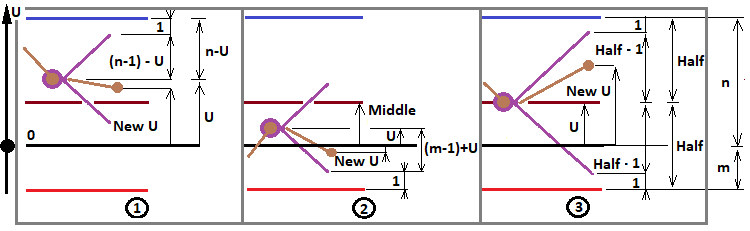This diagram is very appropriate here, since now we determine all possible fractal configurations that may be useful to us for solving most of the problems. According to this diagram, we define three cases for "f":

1. f = ( n - 1 ) - U
2. f = ( m - 1 ) + U
3. f = Half - 1

These three cases appear when the following conditions are met:

1. U > Middle
2. U < Middle
3. U = Middle

Now we need to describe the last two values to be passed to the next fractal level and consider how numbers are collected in the fractal. The last two values are calculated as follows:

• NewP = P * C(f,i) * Pow(p,i) * Pow(1-p,f-i)  — our new chain probability "P" to be passed to the next fractal level
• NewS = S + f = S + (floor(Mid) - 1) — our new "S" to be passed to the next fractal level

Before starting to collect numbers into a common variable, pay attention that numbers should be collected in a similar block - but in this case we only take one step, so we don't need the Bernoulli scheme. The order of the statements is not important; they just should be in the same block. Numbers can only be collected in cases "1" and "2", with some clarifications:

1. U = n - 1
2. U = - ( m - 1 )

For the first case, the previous three values are easier to calculates as we only have one step:

• NewU = U - 1
• NewP = P * p
• NewS = S + 1

For the second case, there is a minor difference:

• NewU = U + 1
• NewP = P * ( 1 - p )
• NewS = S + 1

Based on the generalization of all fractals, each of these fractals is divided into 2 types:

• Fractal calculating the total probability of crossing the upper border of the corridor
• Fractal calculating the total probability of crossing the lower border of the corridor

Each of these types corresponds to one more type of fractal, which is in conjunction with the original:

• Fractal calculating the average number of steps to cross the upper border
• Fractal calculating the average number of steps to cross the lower border

These four fractal types differ in the form of the summed numbers. When collecting the probabilities, we can only add "P*p" and "P*(1-p)". For other two fractals, we need additional variables to pass to the next fractal levels. In these fractals, we use equal-sized steps which are opposite in direction, so their probabilities are either "p" or "1-p". But when "p" is not equal to 0.5, this fact means that these are two different events which can have different characteristics. By characteristics, I mean a set of some random variables that correspond to a given event. One of such values is the position lifetime. There can be any required number of such values, and we can consider them as time if necessary. This part can be simplified after a few easy steps. The numbers to sum will have the following form:

1.  P * p * NewS
2.  P * ( 1 - p ) * NewS

As you can see, the probabilities are multiplied by the number of steps in this completed chain of steps. But this formula only applies when up and down steps are equally probable. In alternative cases, we will need to use two different structures to describe steps up and steps down, or to provide a structure to store both numbers. In the second case, the fractal function will return not a number, but a data container. The container does not require extension. Furthermore, I have provided a container that can store all the required parameters, so that there is no need to describe several functions with a similar code. Instead, I have combined all the functions into one, which can describe all required parameters. The type of the fractal and the task solved by it will depend directly on the input parameters of the function. To extend the concept, the first "S" and its equivalent "NewS" should be replaced with the following values:

1. SU - the final steps up from the selected probability chain
2. SD - the final steps down from the selected probability chain
3. NewSU and NewSD - values to be passed to the next fractal level
4. SU + SD = S

These values should be defined similarly to the definition of "S". When "U > Middle":

• NewSU = SU
• NewSD = SD + 1

When "U < Middle":

• NewSU = SU + 1
• NewSD = SD

Six more values are needed for the final upgrade of the fractal:

1. UpperMidSDown - the total average probable number of steps down before reaching the upper border
2. UpperMidSUp - the total average probable number of steps up before reaching the upper border
3. UpperSummProbability - the probability of crossing the upper border
4. LowerMidSDown - the total average probable number of steps down before reaching the lower border
5. LowerMidSUp - the total average probable number of steps up before reaching the lower border
6. LowerSummProbability - the probability of crossing the lower border

Values "1","2","4","5" indicate the sum of products of the corresponding number of steps and their probability. These values are meaningless as is, but they are components of formulas of useful values which we will discuss further. Values "3" and "6" are the probabilities of hypothesis of crossing two border which form a complete group. Using these values, we can determine a plethora of other things.

### Writing the code to implement a universal fractal

To correctly launch the fractal, we need a function that executes all preparatory operations before fractal launch, after which it correctly launches the fractal based on predefined rules. I have prepared an MQL5-styled implementation of this algorithm:

```Container StartFractal(int m, int n, int s,double p)//preparing all variables and starting the fractal
{
int Minimum;
if ( m <= n ) Minimum=m;
else Minimum=n;
double Middle;
if ( n >= m ) Middle = (m+n)/2.0 - Minimum;
else Middle = -((m+n)/2.0 - Minimum);
double Half = (m+n)/2.0;
return Fractal(Half,Middle,m,n,s,p,0,0,0,1.0);
}
```

After fractal calculation, the function returns our container with all the required data:

```struct Container//a container for collecting all the necessary data about the fractal
{
//values to be summed, for the upper bound
double UpperMidSUp;//the sum of probabilities multiplied by the number of steps up of a specific chain (to cross the upper bound)
double UpperMidSDown;//the sum of probabilities multiplied by the number of steps down of a specific chain (to cross the upper bound
double UpperSummProbability;//the sum of the probabilities (to cross the upper border)
//values to be summed, for the lower border
double LowerMidSUp;//the sum of probabilities multiplied by the number of steps up of a specific chain (to cross the lower border)
double LowerMidSDown;//the sum of probabilities multiplied by the number of steps down of a specific chain (to cross the lower border)
double LowerSummProbability;//the sum of the probabilities (to cross the lower border)

Container()//default constructor
{
UpperMidSUp=0.0;
UpperMidSDown=0.0;
UpperSummProbability=0.0;
LowerMidSUp=0.0;
LowerMidSDown=0.0;
LowerSummProbability=0.0;
}

//
{
c0.UpperMidSUp=c0.UpperMidSUp+c1.UpperMidSUp;
c0.UpperMidSDown=c0.UpperMidSDown+c1.UpperMidSDown;
c0.UpperSummProbability=c0.UpperSummProbability+c1.UpperSummProbability;
c0.LowerMidSUp=c0.LowerMidSUp+c1.LowerMidSUp;
c0.LowerMidSDown=c0.LowerMidSDown+c1.LowerMidSDown;
c0.LowerSummProbability=c0.LowerSummProbability+c1.LowerSummProbability;
}
void operator+=(Container &c) { Summ(this,c); }//operator += overload
};
```

This container includes an overload of the "+=" operator, to combine two identical structures. This part will be used for the main function. Here is the fractal function:

```Container Fractal(double Half, double Middle, int m, int n, int s,double p,int SU,int SD, int U, double P)//Fractal
{
Container C;
///to pass to the next fractal level
int NewU;
int NewSU;
int NewSD;
double NewP;
///

if ( U > Middle && SU + SD < s )//case 1
{
if ( (n-1) - U > 0 )
{
for ( int u=0 ; u <= (n-1) - U; u++ )
{
NewU = -(n-1) + 2*u + 2*U;
NewP = P * (Factorial((n-1) - U)/(Factorial(u)*Factorial((n-1) - U - u))) * pow(p,u)*pow(1.0-p,(n-1) - U - u);
NewSU = SU + u;
NewSD = SD + ((n-1) - U - u);
C+=Fractal(Half,Middle,m,n,s,p,NewSU,NewSD,NewU,NewP);
}
}
if ( (n-1) - U == 0 )
{
NewU = U - 1;
NewP = P * (1.0 - p);
NewSU = SU;
NewSD = SD + 1;
Container ct;

ct.UpperMidSDown=P*p*SD;
ct.UpperMidSUp=P*p*(SU+1);
ct.UpperSummProbability=P*p;

C+=ct;
C+=Fractal(Half,Middle,m,n,s,p,NewSU,NewSD,NewU,NewP);
}
}

if ( U < Middle && SU + SD < s )//case 2
{
if ( (m-1) + U > 0 )
{
for ( int u=0 ; u <= (m-1) + U; u++ )
{
NewU = -(m-1) + 2*u;
NewP = P * (Factorial((m-1) + U)/(Factorial(u)*Factorial((m-1) + U - u))) * pow(p,u)*pow(1.0-p,(m-1) + U - u);
NewSU = SU + u;
NewSD = SD + ((m-1) + U - u);
C+=Fractal(Half,Middle,m,n,s,p,NewSU,NewSD,NewU,NewP);
}
}
if ( (m-1) + U == 0 )
{
NewU = U + 1;
NewP = P * p;
NewSU = SU + 1;
NewSD = SD;
Container ct;

ct.LowerMidSDown=P*(1.0 - p)*(SD+1);
ct.LowerMidSUp=P*(1.0 - p)*SU;
ct.LowerSummProbability=P*(1.0 - p);

C+=ct;
C+=Fractal(Half,Middle,m,n,s,p,NewSU,NewSD,NewU,NewP);
}
}

if ( U == Middle && SU + SD < s )//case 3
{
if ( int(MathFloor(Half))-1 > 0 )
{
for ( int u=0 ; u <= int(MathFloor(Half))-1; u++ )
{
NewU = -(int(MathFloor(Half))-1) + 2*u + U;
NewP = P * (Factorial(int(MathFloor(Half))-1)/(Factorial(u)*Factorial(int(MathFloor(Half))-1 - u))) * pow(p,u)*pow(1.0-p,int(MathFloor(Half))-1 - u);
NewSU = SU + u;
NewSD = SD + (int(MathFloor(Half))-1 - u);
C+=Fractal(Half,Middle,m,n,s,p,NewSU,NewSD,NewU,NewP);
}
}
}

return C;
}
```

The code has been checked in MetaTrader 5 - it works pretty well. This logic can be further expanded, if needed, while there are still a lot of advanced possibilities. But I decided to keep the list of the function input parameters short as for now, because the implemented functionality is quite enough for our purposes. By carefully studying the code, you will see that it fully complies with the mathematical principles outlines above. Although short, this piece of code can work wonders. I believe that the code should contain as much logic and mathematics as possible. This is what we will ultimately need. Even beautiful code is useless if it cannot be used for its intended purpose. In our case, the intended purpose is the applicability for trading. Here is the log as the confirmation: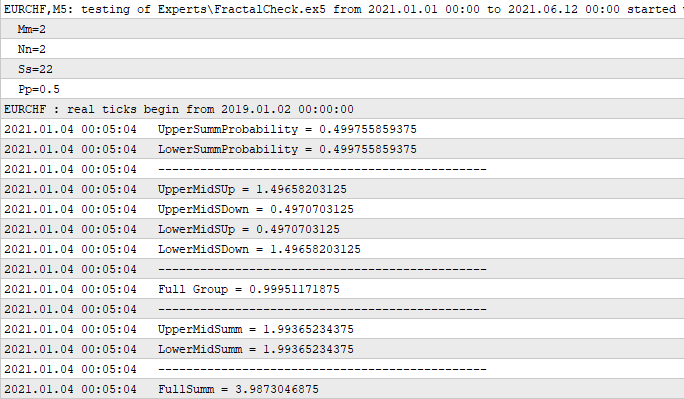In this case, I have created a simple Expert Advisor, which calculates the entire fractal at the first received tick. This calculation is done once, so no further recalculations are needed. The first six numbers are part of the container, while the rest are derived from them. I show here only the most important derivatives, which can assist in understanding that these six variables allow receiving all other variables we may need. For example, take a look at "Full Group". It is named so because the sum of probabilities of two non-overlapping hypotheses of one of the border crosses according to the previous calculation must be equal to one. It is confirmed by our code. This is followed by two identical numbers which are the sums of "1","2" and "3","4". The latter number is the sum of the penultimate numbers - this is the average number of steps which the chain will go through. The reason why I set these input parameters of the set function, where "m" and "n" are equal and symmetric, will be shown later.

### Deriving the first formula based on a symmetric fractal

According to the log result, the average number of steps which the chain will go through tends to "4". The corridor is doubled relative to a unit step. A unit step is if "n" and "m" are set to one. In other words, if we want to calculate the average number of steps in a corridor which consists of smaller corridors (in which case an integer number of smaller corridors fit into a larger one and the new corridor is also symmetric), then we can assume that:

• P[n] = P[n-1] * 2  - is the recursive expression for the new corridor width in steps, based on the width of the previous, smaller corridor, from which the new corridor is composed
• S[n] = S[n-1] * 4 - is the recursive expression for calculating the average number of steps of the new corridor, expressed via the average value of a smaller corridor

if we accept that "P=1" and "S=1", and if we start recursion numbering from index "0", then this recursion can be represented as two very similar series:

• P[n] = 2^n , n = 0 ... + infinity
• S[n] = 4^n = (2^2)^n = (2^n)^2 = P[n]^2

If you look closely at the first series and correctly transform the second series, it turns out that the second series can be expressed using the elements of the first series. In other words, we get the following dependence: S = S(P) = P^2. Now this dependence is only true for the recursive doubling of the channel width. When I saw this formula, I decided to check its applicability for any, arbitrary large number "n" and "m". Logically, in the second step we set "n=3", "m=3" and calculate the same variables. With these input parameters, the average number of steps tends to the number "9". You can check this part yourself, using the above code or using MathCad 15 programs attached below. The same series can be provided for these parameters:

• P[n] = 3^n , n = 0 ... + infinity
• S[n] = 9^n = (3^2)^n = (3^n)^2 = P[n]^2

As you can see, we got the same relationship "S=S(P)=P^2". We could repeat the same for all other possible scenarios related to the interval splitting, but this is not necessary. This fact means that if we know, let's say, the average lifetime of a price inside a symmetric channel, we can calculate the average lifetime of a price inside any other channel. It can be calculated as follows:

• S = S0 * K^2 - the average number of steps of a new corridor
• T = S * T0 - the average lifetime of the new corridor
• T = T0 * K^2- the average lifetime of the new corridor expressed in terms of the average lifetime of another corridor (provided that S0 = 1 )
• S0 - the average number of steps of the old corridor
• T0 - the average lifetime of one step of the old corridor
• P = K * P0  --> K = P/P0 - how many time the new corridor is larger than the old one
• P - the width of the new corridor
• P0 - the width of the old corridor

Now, we can test the hypothesis using MathCad 15. First, let us test the assumptions regarding the quadratic relationship: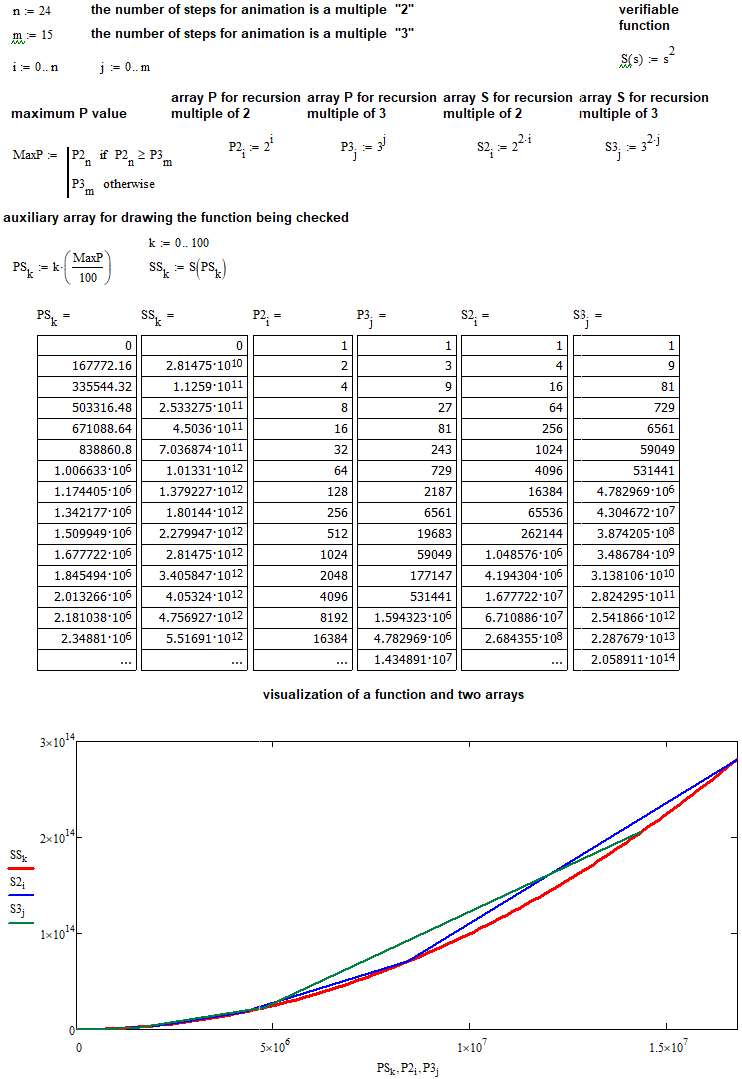Now it should be very clear.

### Evaluating the performance of the derived formula for all positive and real arguments

The formula works for all integer "P". But can it be used for a float "K"? We need to implement a trick to provide a float "K". Suppose we have a price corridor with a known average lifetime, and we have a corridor which fits inside our corridor "N" times, but we don't yet know its average lifetime. Further, we will find it using the same formula. According to this logic:

• T = T0 * N^2 ---> T0 = T / N^2
• T - is the lifetime of our corridor, for which we know the average lifetime
• T0 - is the average lifetime of a smaller corridor, which our corridor is made of

It means that we can find the lifetime of a smaller corridor, which we need for calculating the lifetime of the third corridor with a fractional increase factor. Now that we have found the lifetime of the smallest corridor, we can find its width in points:

• d = P / N

Next, we can calculate how many such corridors fit into a widened corridor, using the following ratio:

• Smin = MathFloor( K * P / d ) = MathFloor( K * N )
• Lim( N --> +infinity ) [ K * N/MathFloor( K * N ) ] = 1

As you can see, the corridor width is reducing, and it does not affect the result. The second line shows a very important ratio that will help to understand what to do next. It shows that when we split the source corridor into as many segments as possible, we can neglect the fractional part that is discarded as a result of the MathFloor function. This is shown by the limit tending to unity. If this inaccuracy is confusing, we can find another value:

• Smax = MathFloor( K * P / d ) + 1 =  MathFloor( K * N ) + 1 = Smin + 1

Now it is clear that the true value of "K * N" is just between "Smin" and "Smax". If "N" tends to infinity, we get two very similar corridors and their average lifetime will tend to be equal, as their sizes only differ by one segment. Thus, the average lifetime of the necessary corridor will be more accurately determined by the arithmetic mean of the average lifetime of these corridors:

• T1 =( T0 * Smin^2 + T0 * Smax^2 ) / 2 =  T0 *( Smin^2 + Smax^2 ) / 2
• T1 - the average lifetime of the corridor which we need to determine

The following figure illustrates my ideas: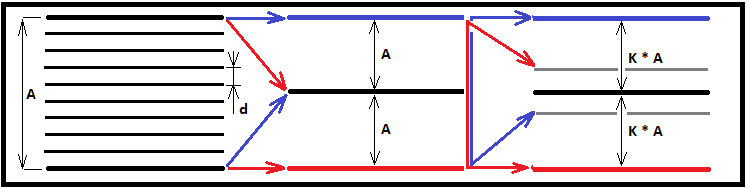Now that we have found an alternative expression for calculating the lifetime of the corridor, we can compare its result with the value of the function for integer "K", substituting float "K" instead. If the resulting values of the two expressions are identical, we can conclude that the found function for integer "K" values is absolutely applicable for any integers and floats in the range of "0 ... +infinity". Let's perform the first check for "N = 1000". I think this splitting will be enough to see the identity of two numbers if there is any: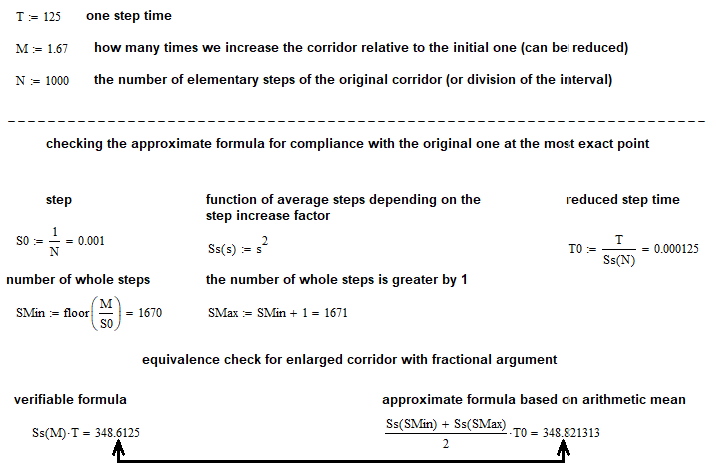As you can see, the two numbers are practically identical. Logically, they should be more identical for larger "N" values. This can also be proved by assuming the following:

• Lim( N --> +infinity ) [  (T0 *( Smin^2 + Smax^2 ) / 2) / ( T * K^2 )  ] = 1

The numerator of this limit is our approximate expression for calculating the average lifetime of the new corridor, and the denominator is an expression that presumably accurately describes the same value. I have created a simple function performing the same calculations as in the previous screenshot. But this time it is applied to the entire range of the "N" number starting with "1". Now let's view the program execution result: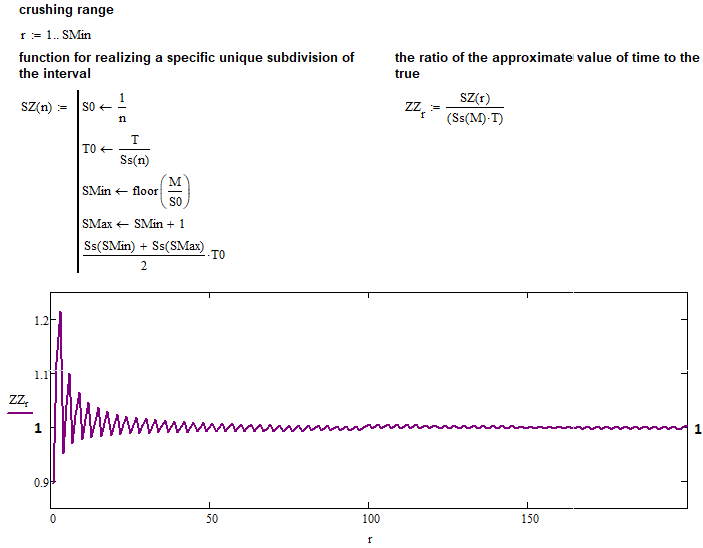All assumptions are fully confirmed: the function that we found for integer "K" is absolutely applicable for any positive "K". Now we have a single, very useful function which can be used as the basis for further actions, for example as the basis for further mathematics for describing the entire universal fractal.

### Advanced fractal developed from the universal fractal

As a useful and additional example of further application of a universal fractal, we can take a one-border fractal, with, say, "n=1", "m ---> +infinity", "s = m+1", "p=0.5". So far, we are considering fractals with equal-probability steps in both directions, which is applicable only to random walk. But this fractal provides all the possibilities. In order to move to a deeper analysis of such a complex structure, it is first necessary to consider the fundamentals. Furthermore, at this initial step we obtain useful formulas and can make fundamental conclusions regarding these fractal processes. I tested the fractal with different "s" values and got the following data:

• s = 22 , FullSumm = 2.868 , UpperSummProbability = 0.831
• s = 32 , FullSumm = 3.618 , UpperSummProbability = 0.860
• s = 42 , FullSumm = 4.262 , UpperSummProbability = 0.877
• s = 45 , FullSumm = 4.499 , UpperSummProbability = 0.882

A further increase in the number of permissible steps leads to the computation time singularity, in other words, the computation time increases so much that it would require hours or even days. But if you look at the speed at which the average probability sum increases, you can see that it is not possible to evaluate the convergence of this series using this type of fractal. But based on the previously derived formula, we can evaluate convergence using a different yet very useful fractal type. This fractal can also assist in calculating the time for a very popular and profitable strategy entitled "Carry trade". First, I'll show a figure, and then I'll explain it: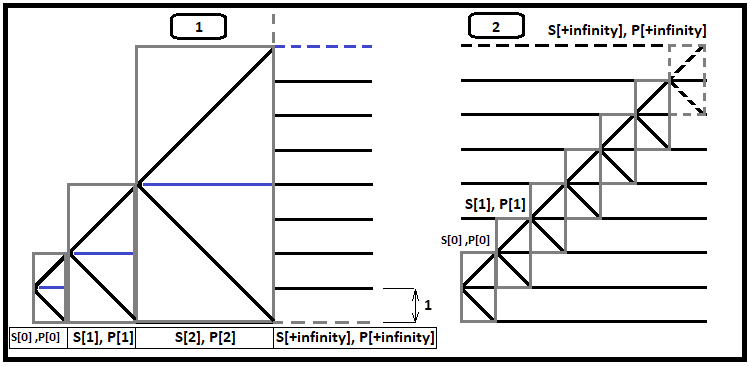Imagine that the pricing process starts one step from the border, no matter where the border is, up or down. The figure above shows an example with a lower border, as it is easier to perceive. Take a closer look at the first fractal. Each gray box contains two scenarios for further events:

1. Price reaches the upper edge of the box
2. Price reaches the lower edge of the box

When the price reaches the upper border of the corridor, a new larger fractal automatically begins at this point, and so on. If we consider this process in the same way as in the universal fractal, we will again see the probability chains. But now our formula tells which number of steps that will be made in a symmetric corridor, based on how many steps fit into the corridor (now we see that a step is a smaller corridor which fits into the original one).

Now, you do not need to consider the entire fractal to calculate the average number of steps. Instead, apply the derived formula to each of the fractals. The step here is not the same value, but it is made when we reach the upper or lower bound of the next nested fractal. Based on this, we can create probability chains which will be very simple. The probability of reaching the border at the very first fractal P is equal to 0.5. It means there is a second possible case when we have to create the next fractal hoping that the price will reach the border. All these events are nested, and all such chains form a complete group.

The probability of reaching the bound at the second fractal P is equal to the previous probability multiplied by 0.5. This process can be continued indefinitely. The average number of steps can be determined using the derived formula and chain probabilities. To do this, we first define a formula for the probability of each individual chain, taking into account that the average number of steps to cross the upper bound and to cross the lower bound is equal. It turns out that:

• PUp = PDown = P - the ratio showing that the probabilities of touching the upper and lower bounds of a fractal are equally probable for all bounds of all nested fractals
• P[j] = 0.5^(j+1) , j = 0 ... + infinity- the probability that chain j will happen
• S[i] = S[i-1] + P[i] * ( S[i-1]/P[i-1] + F(D[i]) ),  i = 1... + infinity - a recurring formula to calculate the total averagely probable number of steps for all fractal levels (while S = 1*0.5 = 0.5)
• F(K) = K^2 - our derived formula for calculating the average number of steps
• D(i) = 2^i - how many steps fit in the next fractal level
• S[i-1]/P[i-1] - the average number of steps in the remaining unaccounted branch, provided that the current branch has occurred (because in addition to the current nested fractal, it is necessary to take into account all steps that happened before)

The second fractal is actually identical to the first one, i.e. the probabilities of their chains (the P[] array) are identical. Why do we need it? Suppose we have the "Carry Trade" strategy. We have two accounts, a swap account and a swap-free account to lock positions with a positive swap. We also need a formula to calculate the average profitable position holding time. This average holding time directly stems from the average number of steps formula. I will not cover this issue in detail in this article. I just want to show the importance of the math. This topic will be discussed in detail later. Now, let's define a formula for the average probability steps for the second fractal:

• S[j] = S[j-1] + P[j] * ( S[i-1]/P[i-1] + F(1) ) - a recurring formula to calculate the total averagely probable number of steps for all fractal levels (while  S = 1*0.5 = 0.5)

In this case, this formula is only a special case of the precious one because in the second fractal K=1, always, for all fractal levels. Let's find out what the limits of the sums of these quantities are equal to for both fractals: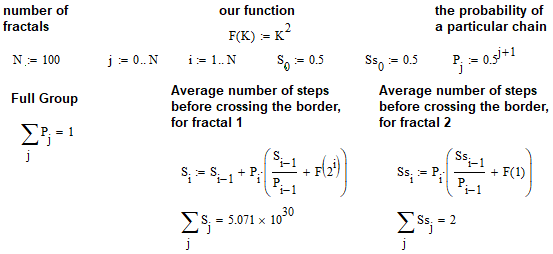The first series diverges, which means that when there is no upper bound and the trading is endless, the average time is equal to infinity. In the second case we get a clear limit equal to 2. It means that if we open a position with a positive swap, on the average we will need to close this position after two steps (thus the average position holding time will be equal to 2*T, where T is the average position holding time provided that we close the position when it reaches one of the bounds). In the second and simplest case, we simply close both positions on both accounts, even if the swap account shows a positive number. Obviously, the first option is much more attractive, but its implementation would require a quick and smooth withdrawal and deposit on both accounts. If this option is not possible, we will need to use a classic option, which generates less profits but has greater stability.

### Summarizing the results

In the second part of the article series, we achieved very interesting results, including results for practical application in trading. What's more important, it is now clear that the sphere of possible application of fractals is huge. What we have done in the article:

• Defined clear mathematical rules for building a universal fractal
• Created a working MQL5-style code based on the stated mathematical principles
• Successfully checked the principles using two platforms, MetaTrader 5 and MathCad 15
• Using the algorithms, obtained the first formula for calculating the lifetime of an arbitrary corridor
• Tested and validated the formula using programming for all possible cases
• Obtained a new type of a faster fractal, based on the obtained formula
• The resulting allowed us to speed up calculations and to determine what couldn't be determined by a universal fractal
• Touched upon a special case of using an advanced fractal for swap trading problems
• Obtained tools for further development of the theory

Also I'd like to note that my toolbox has greatly expanded. Now we can analyze fractals with different-probability steps (trend situations and situations related to trading statistics analysis). Once again, please note that in this article we only considered cases where "p = 1 - p = q = 0.5". This means that all calculations are only applicable to the situations that describe random walk. Thus, there are much more potential possibilities.

Below is the formula which was obtained after studying the fractal. Once again, I will briefly explain it:

1. S = K^2 - the average number of steps of the new corridor, based on the fact that a step is equal to another corridor
2. T = S * T0 =  T0 * K^2- average lifetime of an unknown corridor
3. T0 - average lifetime of a known corridor
4. P = K * P0  --> K = P/P0 - how many times the known corridor is larger than the unknown one
5. P - the width of the corridor with an unknown lifetime
6. P0 - the width of the known corridor

By substituting 4 into 1, we can express the average number of steps that will be in the unknown corridor through the known values, and substituting 1 into 2 we get the average lifetime of this corridor, which is calculated based on the known values:

• T0, P0, P

• Why do we need formulas if we can collect trading statistics from the MetaTrader 5 strategy tester?

Response:

• Well, it is not always possible to collect statistics as not all financial symbols have sufficient trading history required for such assessments. This is impossible for large corridors, as one could select a corridor that has never crossed its borders. Using corridors with smaller width and the formula, we can obtain the data which cannot be obtained from statistics. Furthermore, this kind of math gives you unrivaled accuracy and flexibility. There are much more other advantages.

### Conclusion

In this article, I tried to describe the calculation logic, which can be used to conduct further research regarding pricing processes. So far there is not much for practical application. But I am sure that we can study all the varieties of the universal fractal and obtain possible formulas for describing asymmetric and one-border price corridors. In the next article, I will continue to research the universal fractal and will try to present all the results as simple formulas. Also, I will include new, very interesting mathematics that will allow using the obtained formulas not only for pricing processes, but also for describing backtests and trading signals. This will enable us to absolutely accurately analyze any strategy in terms of time and probabilities, which is ultimately the most important aspect of trading.

### References

• #### Combinatorics and probability theory for trading (Part I): The basics

Translated from Russian by MetaQuotes Ltd.
Original article: https://www.mql5.com/ru/articles/9511

Attached files |
Materials.zip (270.97 KB)

#### Other articles by this author

Last comments | Go to discussion (1)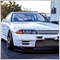| 6 Oct 2021 at 18:07
ok i understand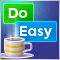Graphics in DoEasy library (Part 82): Library objects refactoring and collection of graphical objects
In this article, I will improve all library objects by assigning a unique type to each object and continue the development of the library graphical objects collection class.Graphics in DoEasy library (Part 81): Integrating graphics into library objects
It is time to start the integration of the already created objects into the previously created library objects. This will ultimately endow each library object with its own graphical object allowing users to interact with the program.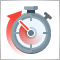Dealing with Time (Part 1): The Basics
Functions and code snippets that simplify and clarify the handling of time, broker offset, and the changes to summer or winter time. Accurate timing may be a crucial element in trading. At the current hour, is the stock exchange in London or New York already open or not yet open, when does the trading time for Forex trading start and end? For a trader who trades manually and live, this is not a big problem.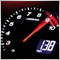Better programmer (Part 05): How to become a faster developer
Every developer wants to be able to write code faster, and being able to code faster and effective is not some kind of special ability that only a few people are born with. It's a skill that can be learned, that is what I'm trying to teach in this article.